[Go to Previous] [Go to Next]

Formulas

McIDAS-V provides techniques to make computations with data sources and to display the results. The simplest technique is using McIDAS-V formulas, which are one-line mathematical expressions. The steps for computing and displaying the output of a McIDAS-V formula are:

• Defining a McIDAS-V formula by name, description, and mathematical formula.
• Saving the formula.
• Selecting the display type.
• Selecting which data to use in the formula.
You can make formulas more powerful by writing methods (subroutines) in the Jython computer language, which is described in Jython Methods.

Once you define a McIDAS-V formula, it is saved and will appear in future sessions of McIDAS-V.

To create a formula, select the Tools->Formulas->Create Formula menu item in the Main Display window. This opens the Formula Editor dialog window used to define a formula: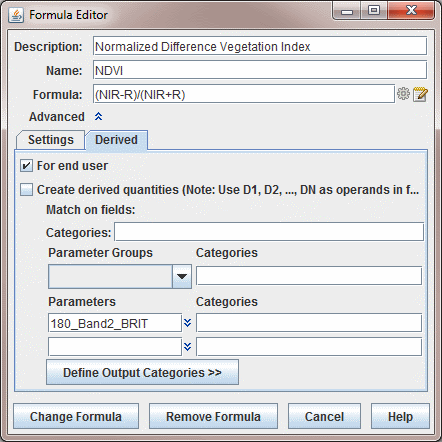Image 1: Formula Editor Dialog
Properties
• Description - Represents the name of the formula that will be written in the Field Selector tab of the Data Explorer.
• Name - Sets the name of the formula to be used in the Parameter Defaults. This allows for settings such as color tables to be automatically assigned to the display when the formula is evaluated. For example, if T is entered as the Name, the displays created by this formula would use the Temperature color table.
• Formula - Represents the formula that will be evaluated.

The formula itself can use common mathematical operators much as +, -, * for multiply, / for divide, and ** for power. Other Jython operations such as sqrt(a), max(b), pow(a,b), and sin(c) are available. The Jython Methods section of the User's Guide explains how to write complete multi-line functions or Jython methods that you can call in one-line formulas.

The variable names in the formula definition, such as "NIR" in the figure above, are operands and do not need to exactly match any particular parameter name in any data source; the association is made later, in the parameter selection step.

Do not use spaces in formula variable names, such as "wind speed." The formula is an expression in the Jython language. Each variable name must start with a letter.

It is best to use variable names that suggest the parameter data they represent, so that later you can easily remember what the variables mean. Use names like Temp500m, RelHum_surf, absvort, sst_jan, density, or whatever makes sense to you. If you use a variable names like V1 and V2, then later when the formula requests which real parameter name goes with which variable name you may be puzzled which is which.

Variable names can have extra "syntactic sugar" attached to them that McIDAS-V uses for a variety of purposes. The "sugar" consists of a set of name/value pairs contained in brackets ("[...]") after the variable name.

For example, normally variables are used to select and define data choices. You can also have variables that are simple text input from a user. For example, evaluating the following expression:

```   someProcedure(someValue[isuser=true])
```

will result in a dialog box being shown to allow for the user to enter a text value (e.g., a number) for the variable someValue.

You can provide default values with:

```   someProcedure(someValue[isuser=true,default=some default])
```

An extension from the previous example, a formula can be created without using a pre-defined procedure from the Jython Library. For example, a formula can be created that will allow the user to divide the values of a field by a specific value. This can be done as follows, by specifying that the value is a float (the value could also be specified as an integer by specifying 'int' in place of 'float'):

`   field/float(value[isuser=true,default=some default])`

You can specify the types of data that can be selected for a variable with the categories name. e.g.:

```   someProcedure(someDataValue[categories=param:ALTITUDE])
```

If you want multiple data categories, separate them with a ";":

```   someProcedure(someDataValue[categories=param:ALTITUDE;param:elevation])
```

If you only want to select 3D grids do:

```   someProcedure(someDataValue[categories=GRID-3D-*])
```

If you only want to select 3D or 2D grids do:

```   someProcedure(someDataValue[categories=GRID-3D-*;GRID-2D-*"])
```

You can also specify a regular expression pattern that is used to pre-select the first data choice whose description matches the pattern.

```   someProcedure(someValue[pattern=some_pattern])
```

A formula can be created that allows for multiple line text input by specifying rows= in the formula. For example:

`   someProcedure(someValue(isuser=true,rows=4))`

A formula can prompt the user with a menu of choices with type=choice in the formula. For example:

`   someProcedure(someValue(isuser=true,type=choice,choices=lt;gt))`

A formula can prompt the user with a Field Selector window that allows for selecting multiple fields at once with multiple=true in the formula. For example:

`   someProcedure(someValue(multiple=true))`

In the advanced section you can enter a description of the formula, its group or category, and you can define what types of displays are applicable for the given formula (the default is to be able to use all displays).

The group is used to display the formula in the Field Selector window and can be any alphanumeric text including spaces, numbers, and punctuation characters. Hyphens ("-") define sub-categories.

To save the formula, click on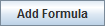. A new entry appears in the selector panel. By right clicking on the formula entry in the Field Selector window you can edit the formula. If you edit a native formula and save it, the original formula will still be listed, but the modified formula will also be listed. To remove a formula, click on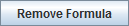in the pull down menu. You can also right click on a formula and select Remove Formula.

For information about the Derived tab, see Derived Data.

Different data sources and automatic navigation

McIDAS-V hides much of the computational complexity. You can write a simple formula such as (CT*1.8 + 32.0) - dewptF, and McIDAS-V will automatically apply the calculation to every element in large 3D grids referred to as CT and dewptF. No loops over grid points are needed; and you don't need to know anything about how the grid is defined, mapped on the Earth, or the data format. You can even use different sources for the individual parameters. For example, CT can come from a NAM model output grid and dewptF from your own local weather model. The two grids don't need to have the same grid point locations nor the same areal coverage. The results are computed in the area of overlap on the grid points locations of the first grid. Interpolation is automatically applied where needed.

Creating a Display with a Formula

Using a formula as the data when creating a display is just like using any other data. You select the formula entry in the Field Selector window, and the list of applicable displays will be shown and you create the display.

When you create a display, McIDAS-V needs to know what actual data should be bound to the operands within your formula. A Field Selector window will appear that allows you to select the actual data for each operand. You can select parameters from more than one data source for use in a single formula, for example, you can pick the same parameter from data sources of different times to compute a time difference in the parameter.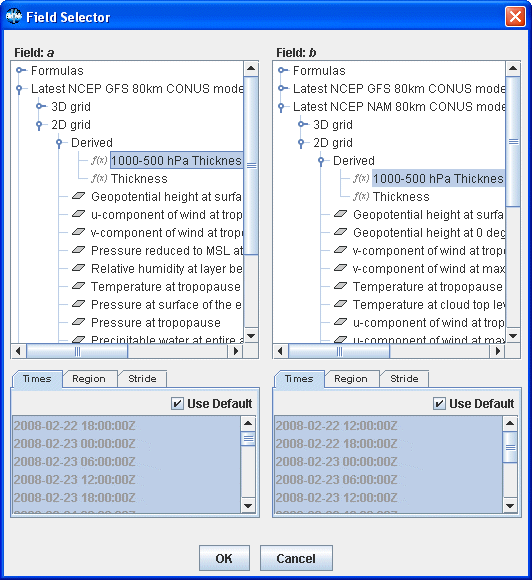Image 2: Field Selector Window

Open the data source to see the names of parameters available. Click on the parameter needed for each variable. Then click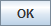. The result of the formula is computed for every data point in the source, and the result shown in the Main Display window.

Note that other windows may appear when evaluating a formula. For example, a Select Input window may be used if the formula prompts the user for a numerical value. This Select Input window may involve a combination of text fields or drop-down menus.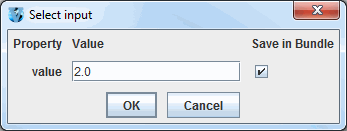Image 3: Select Input Window

[Go to Previous] [Go to Next]